## Описание презентации THE DIHYBRID CROSS Studying the inheritance of по слайдам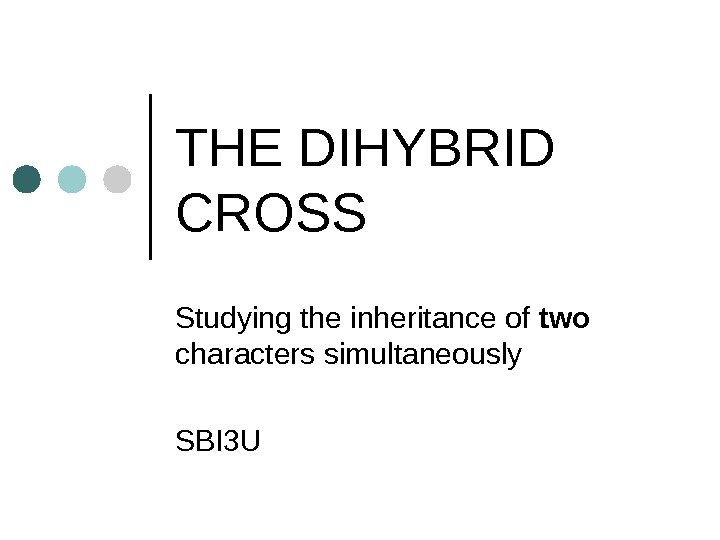THE DIHYBRID CROSS Studying the inheritance of two characters simultaneously SBI 3 U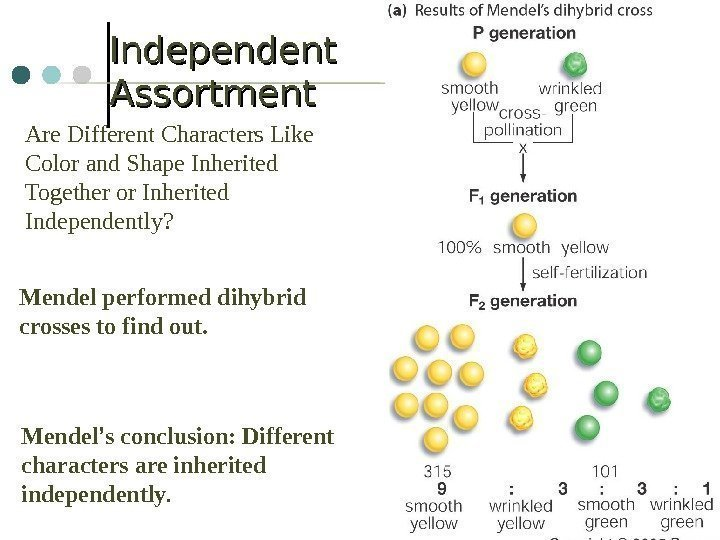Independent Assortment Are Different Characters Like Color and Shape Inherited Together or Inherited Independently? Mendel performed dihybrid crosses to find out. Mendel ’ s conclusion: Different characters are inherited independently.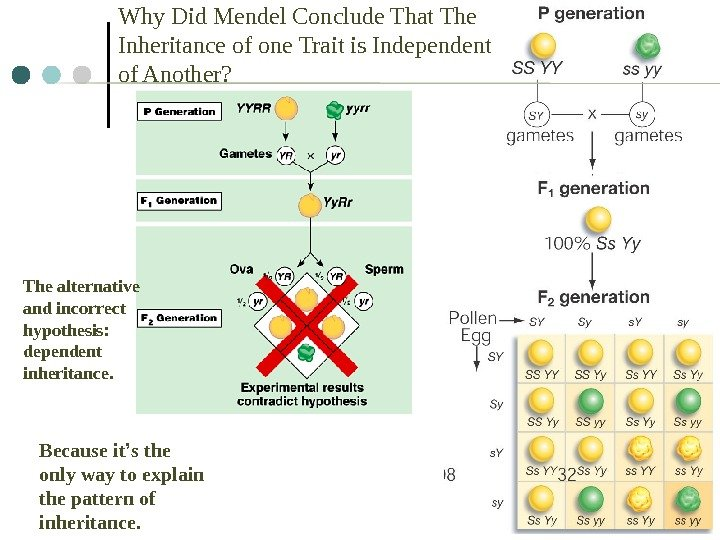Why Did Mendel Conclude That The Inheritance of one Trait is Independent of Another? The alternative and incorrect hypothesis: dependent inheritance. Because it ’ s the only way to explain the pattern of inheritance.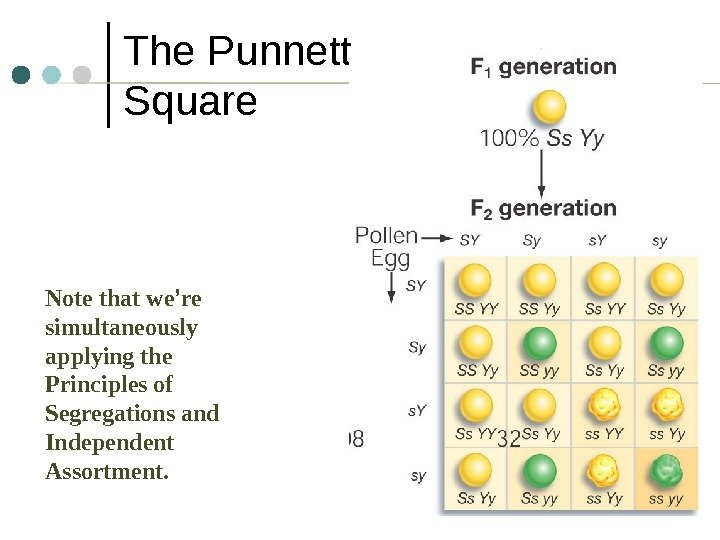4 The Punnett Square Note that we ’ re simultaneously applying the Principles of Segregations and Independent Assortment.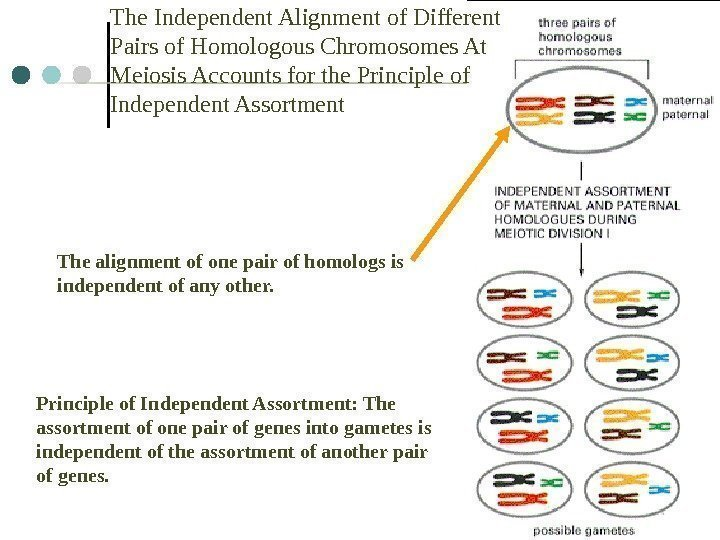5 The Independent Alignment of Different Pairs of Homologous Chromosomes At Meiosis Accounts for the Principle of Independent Assortment The alignment of one pair of homologs is independent of any other. Principle of Independent Assortment: The assortment of one pair of genes into gametes is independent of the assortment of another pair of genes.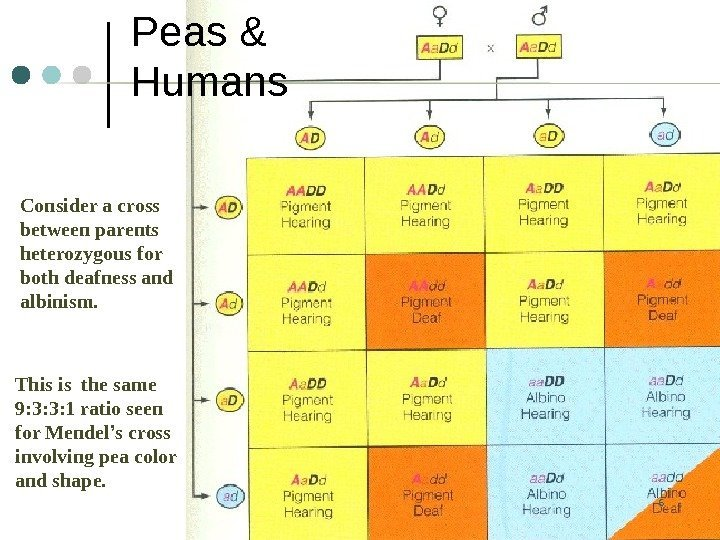6 Peas & Humans Consider a cross between parents heterozygous for both deafness and albinism. This is the same 9: 3: 3: 1 ratio seen for Mendel ’ s cross involving pea color and shape.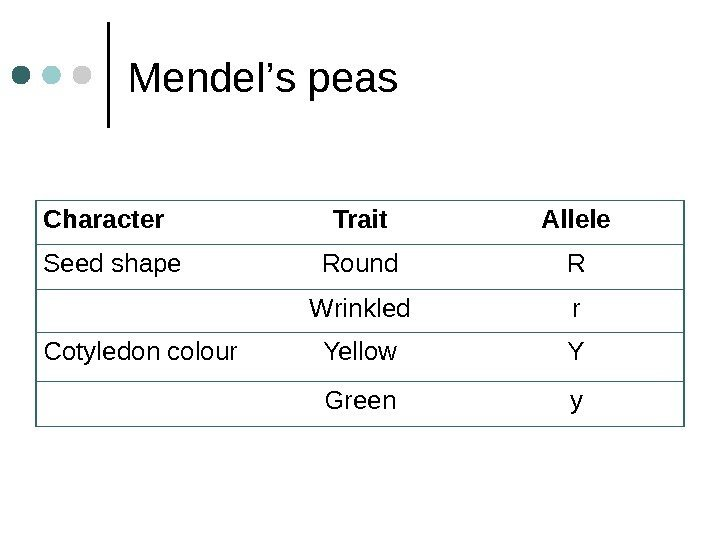Mendel ’ s peas Character Trait Allele Seed shape Round R Wrinkled r Cotyledon colour Yellow Y Green y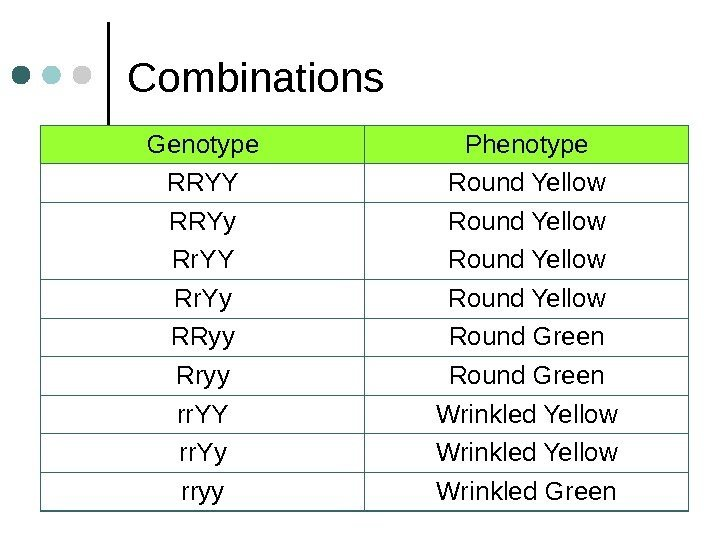Combinations Genotype Phenotype RRYY Round Yellow RRYy Round Yellow Rr. YY Round Yellow Rr. Yy Round Yellow RRyy Round Green Rryy Round Green rr. YY Wrinkled Yellow rr. Yy Wrinkled Yellow rryy Wrinkled Green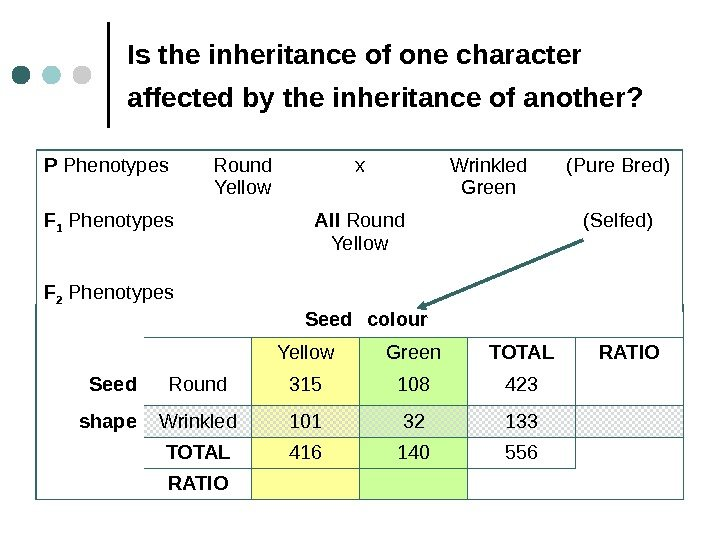Is the inheritance of one character affected by the inheritance of another? P Phenotypes Round Yellow x Wrinkled Green (Pure Bred) F 1 Phenotypes All Round Yellow (Selfed) F 2 Phenotypes Seed colour Yellow Green TOTAL RATIO Seed Round 315 108 423 shape Wrinkled 101 32 133 TOTAL 416 140 556 RATIO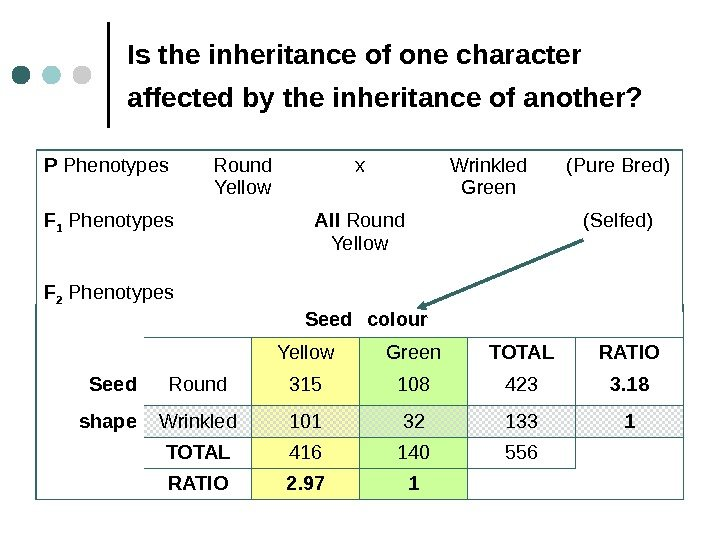Is the inheritance of one character affected by the inheritance of another? P Phenotypes Round Yellow x Wrinkled Green (Pure Bred) F 1 Phenotypes All Round Yellow (Selfed) F 2 Phenotypes Seed colour Yellow Green TOTAL RATIO Seed Round 315 108 423 3. 18 shape Wrinkled 101 32 133 1 TOTAL 416 140 556 RATIO 2.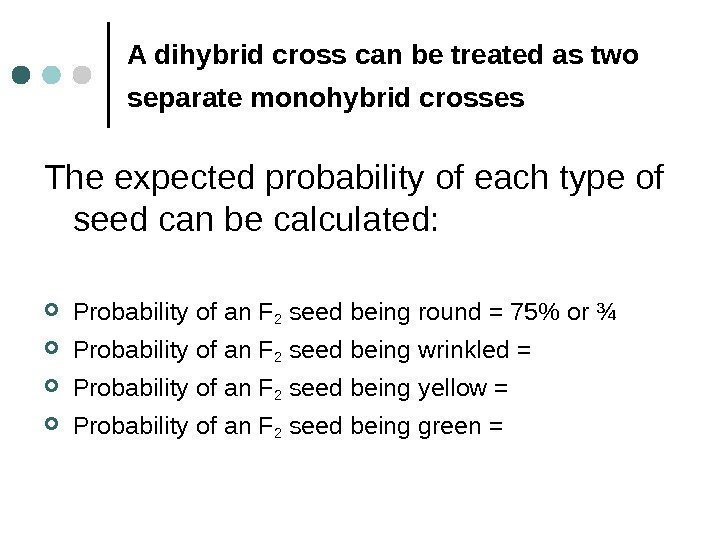A dihybrid cross can be treated as two separate monohybrid crosses The expected probability of each type of seed can be calculated: Probability of an F 2 seed being round = 75% or ¾ Probability of an F 2 seed being wrinkled = Probability of an F 2 seed being yellow = Probability of an F 2 seed being green =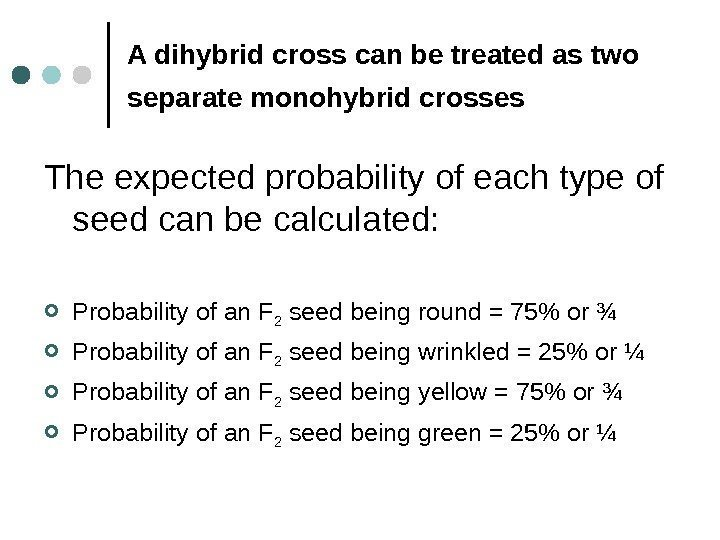A dihybrid cross can be treated as two separate monohybrid crosses The expected probability of each type of seed can be calculated: Probability of an F 2 seed being round = 75% or ¾ Probability of an F 2 seed being wrinkled = 25% or ¼ Probability of an F 2 seed being yellow = 75% or ¾ Probability of an F 2 seed being green = 25% or ¼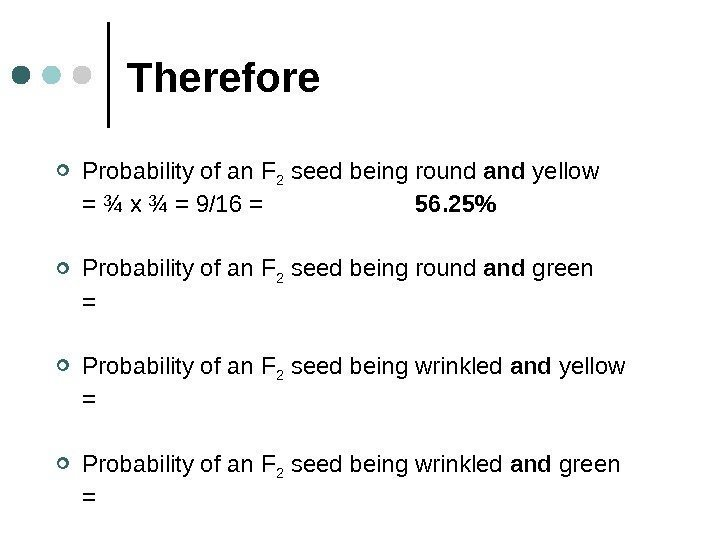Therefore Probability of an F 2 seed being round and yellow = ¾ x ¾ = 9/16 = 56. 25% Probability of an F 2 seed being round and green = Probability of an F 2 seed being wrinkled and yellow = Probability of an F 2 seed being wrinkled and green =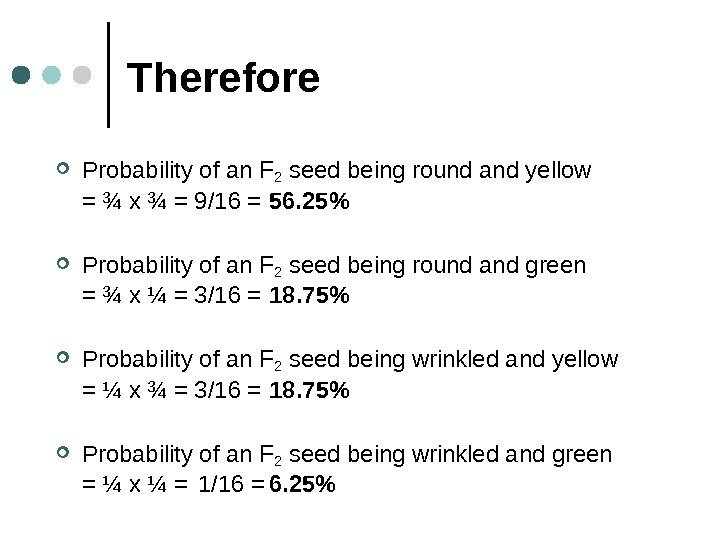Therefore Probability of an F 2 seed being round and yellow = ¾ x ¾ = 9/16 = 56. 25% Probability of an F 2 seed being round and green = ¾ x ¼ = 3/16 = 18. 75% Probability of an F 2 seed being wrinkled and yellow = ¼ x ¾ = 3/16 = 18. 75% Probability of an F 2 seed being wrinkled and green = ¼ x ¼ = 1/16 = 6. 25%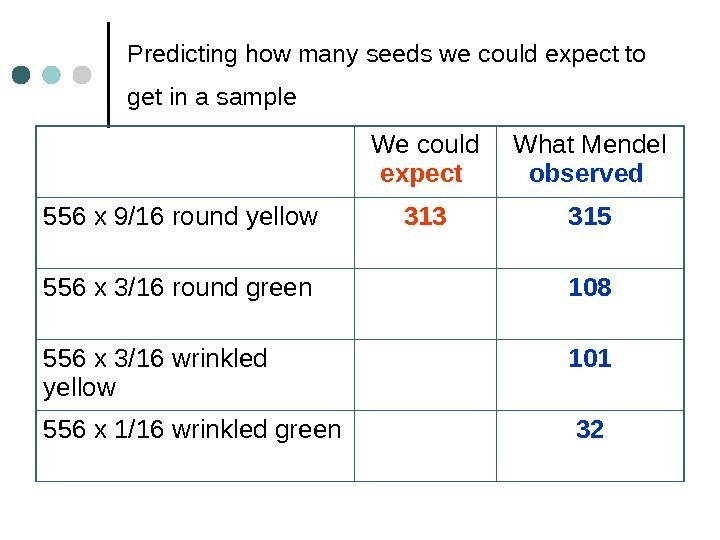Predicting how many seeds we could expect to get in a sample We could expect What Mendel observed 556 x 9/16 round yellow 313 315 556 x 3/16 round green 108 556 x 3/16 wrinkled yellow 101 556 x 1/16 wrinkled green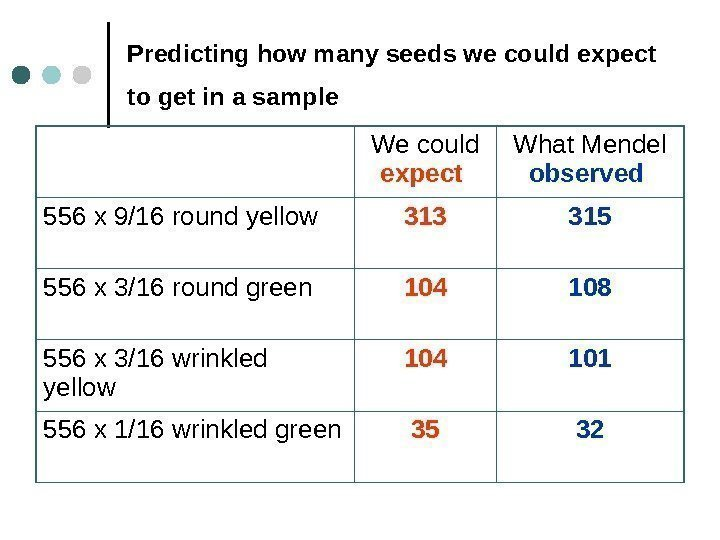Predicting how many seeds we could expect to get in a sample We could expect What Mendel observed 556 x 9/16 round yellow 313 315 556 x 3/16 round green 104 108 556 x 3/16 wrinkled yellow 104 101 556 x 1/16 wrinkled green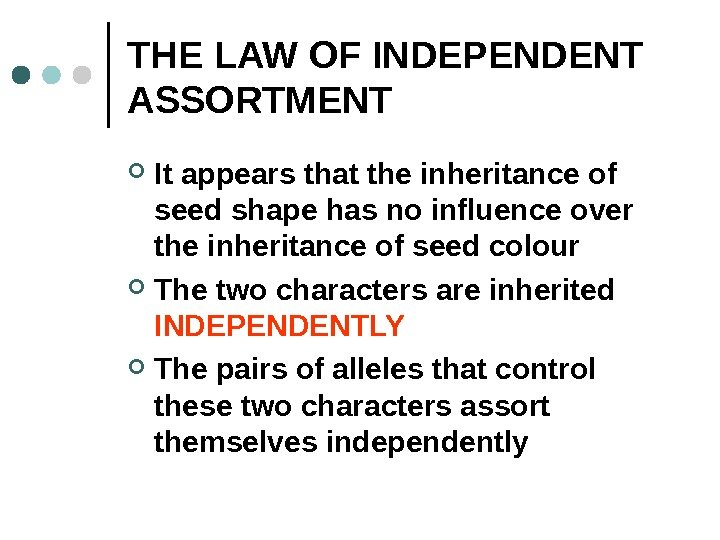THE LAW OF INDEPENDENT ASSORTMENT It appears that the inheritance of seed shape has no influence over the inheritance of seed colour The two characters are inherited INDEPENDENTLY The pairs of alleles that control these two characters assort themselves independently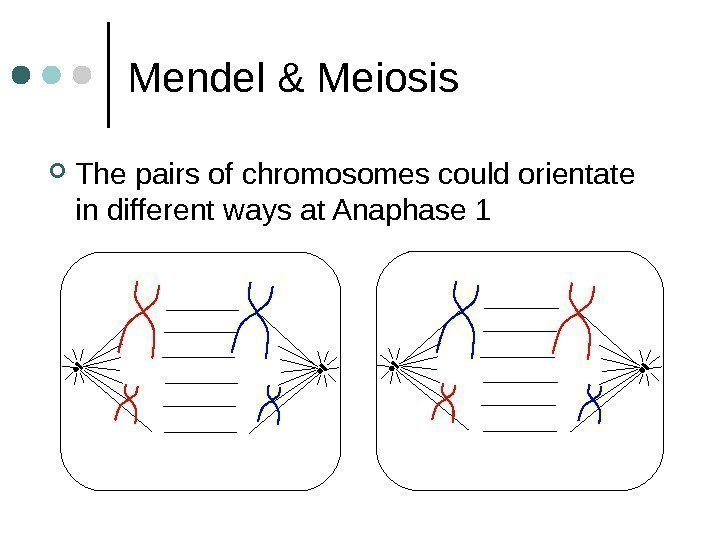Mendel & Meiosis The pairs of chromosomes could orientate in different ways at Anaphase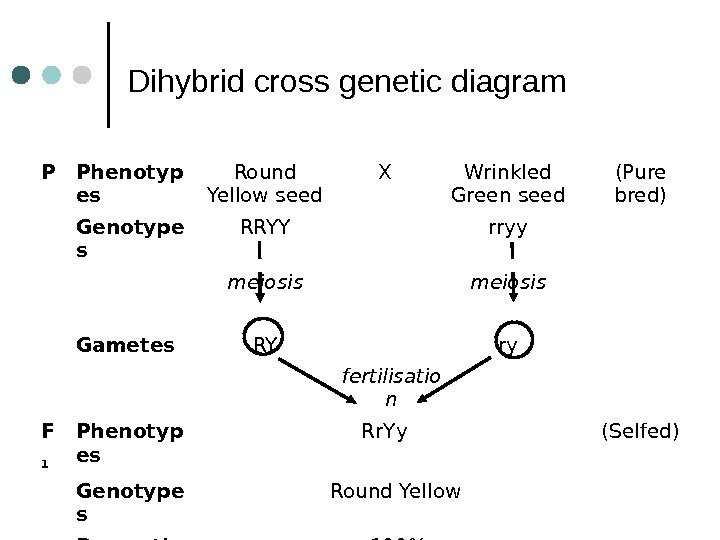P Phenotyp es Round Yellow seed X Wrinkled Green seed (Pure bred) Genotype s RRYY rryy meiosis Gametes RY ry fertilisatio n F 1 Phenotyp es Rr. Yy (Selfed) Genotype s Round Yellow Proportio ns 100%Dihybrid cross genetic diagram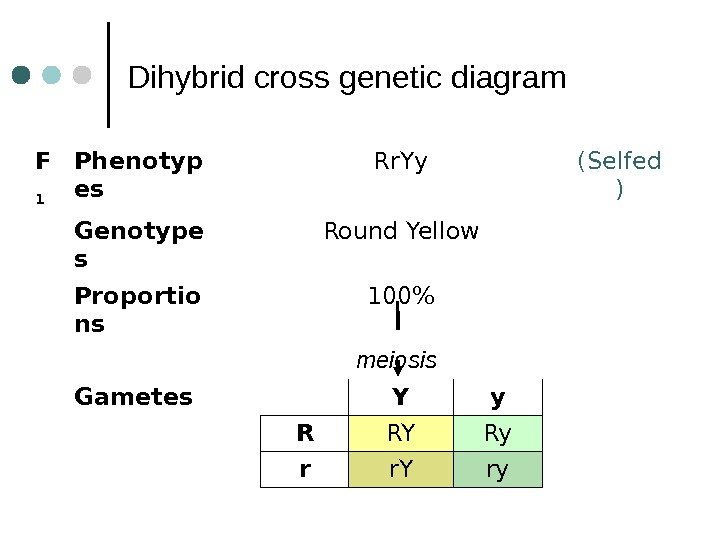F 1 Phenotyp es Rr. Yy (Selfed ) Genotype s Round Yellow Proportio ns 100% meiosis Gametes Y y R RY Ry r r. Y ry. Dihybrid cross genetic diagram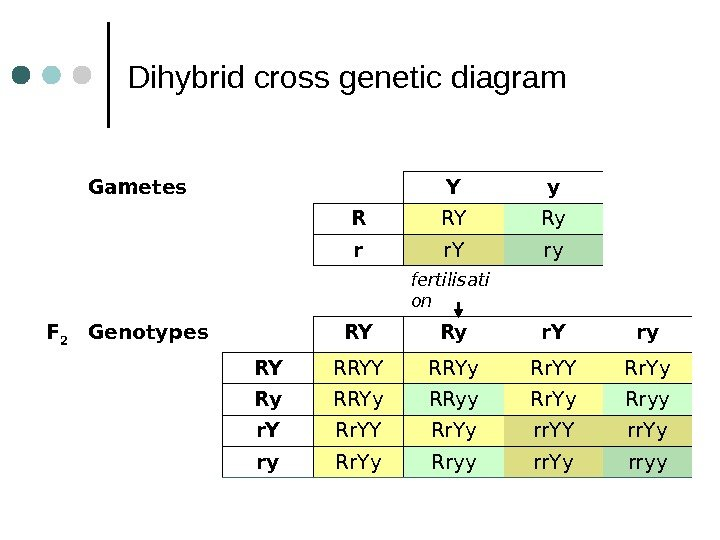Gametes Y y R RY Ry r r. Y ry fertilisati on F 2 Genotypes RY Ry r. Y ry RY RRYy Rr. YY Rr. Yy Ry RRYy RRyy Rr. Yy Rryy r. Y Rr. Yy rr. YY rr. Yy ry Rr. Yy Rryy rr. Yy rryy. Dihybrid cross genetic diagram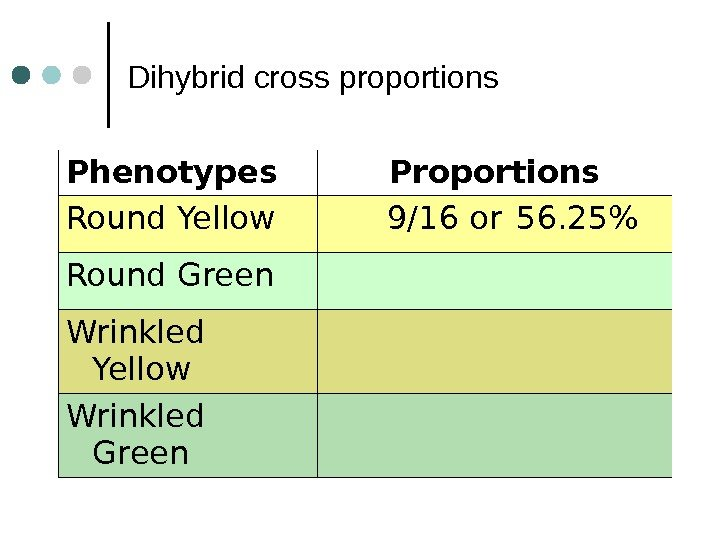Dihybrid cross proportions Phenotypes Proportions Round Yellow 9/16 or 56. 25% Round Green Wrinkled Yellow Wrinkled Green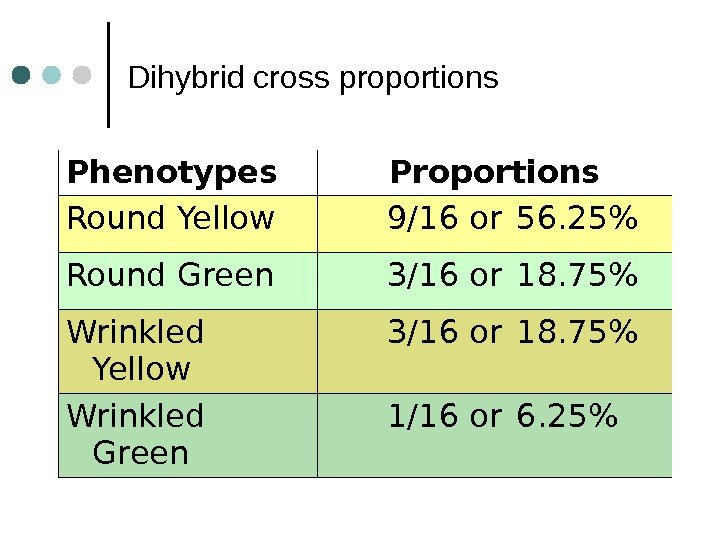Dihybrid cross proportions Phenotypes Proportions Round Yellow 9/16 or 56. 25% Round Green 3/16 or 18. 75% Wrinkled Yellow 3/16 or 18. 75% Wrinkled Green 1/16 or 6. 25%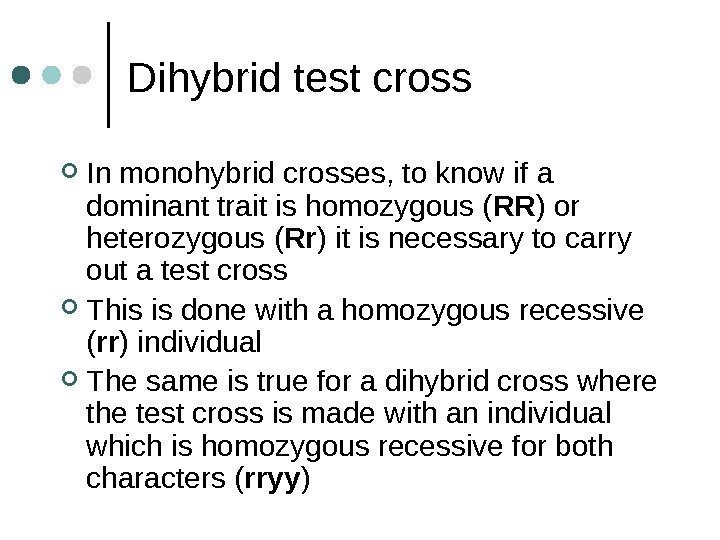Dihybrid test cross In monohybrid crosses, to know if a dominant trait is homozygous ( RR ) or heterozygous ( Rr ) it is necessary to carry out a test cross This is done with a homozygous recessive ( rr ) individual The same is true for a dihybrid cross where the test cross is made with an individual which is homozygous recessive for both characters ( rryy )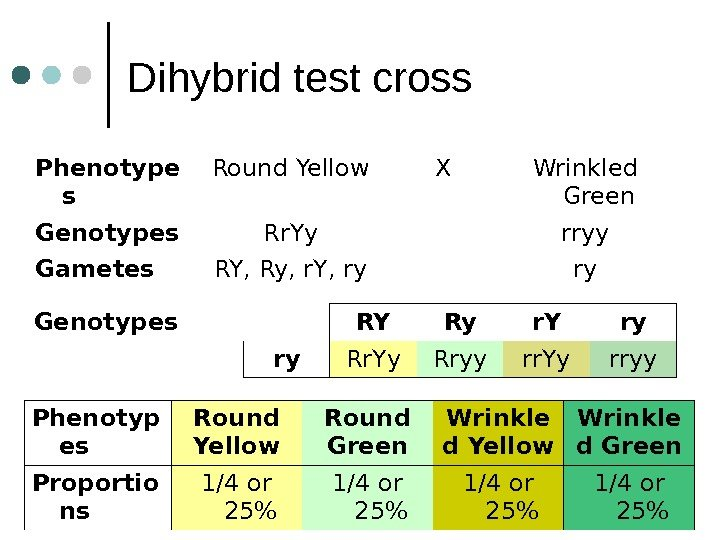Dihybrid test cross Phenotype s Round Yellow X Wrinkled Green Genotypes Rr. Yy rryy Gametes RY, Ry, r. Y, ry ry Genotypes RY Ry r. Y ry ry Rr. Yy Rryy rr. Yy rryy Phenotyp es Round Yellow Round Green Wrinkle d Yellow Wrinkle d Green Proportio ns 1/4 or 25%